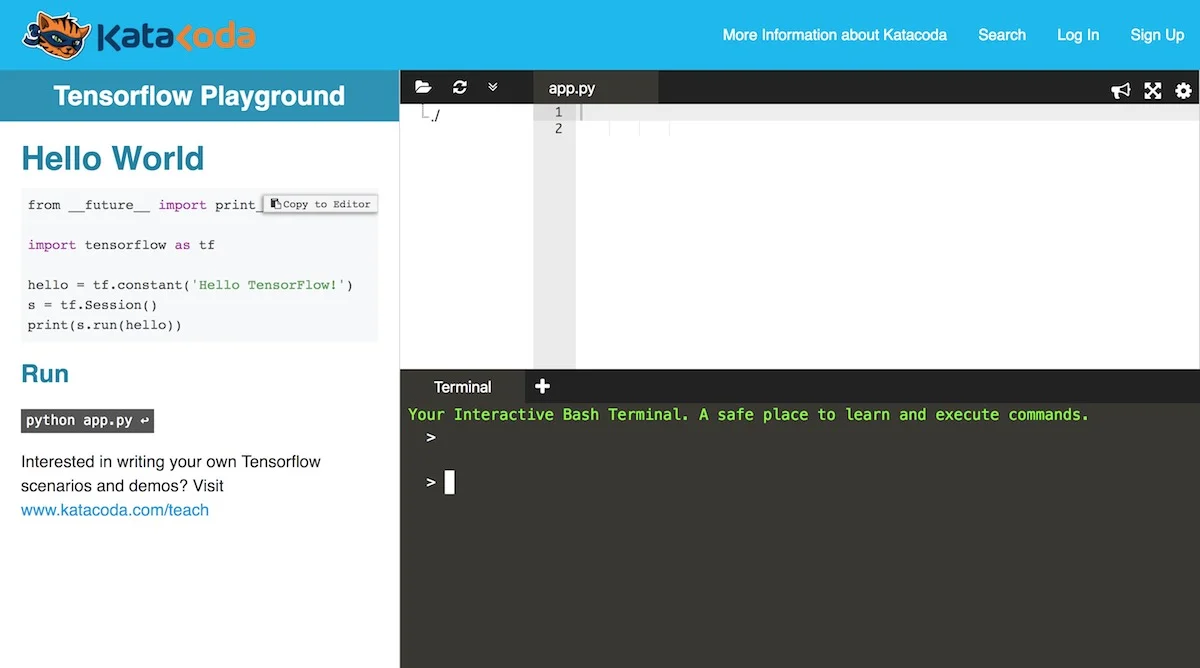# Getting Started with TensorFlow – No Setup Required

290Getting started with TensorFlow can be a bit tricky if you don’t have the right software and/or hardware environment. Here is a super-easy way around that.

I was at a GDG (Google Developer Group) Cloud meetup TensorFlow Hack Session to get more into TensorFlow programming, when the first topic/stumbling block was: “How do we get started?”

We were working in small groups and the main issue was whether we should install the languages (mostly Python) locally and have TensorFlow run locally as well, or whether we should set up some cloud-based approach to be able to write and explore first code samples.

A young “TensorFlow pro” was introduced to us by Yaz (the organiser, a cool Canadian TensorFlow googler) to help us with initial questions. I will credit him as soon as I meet him again and capture his name and contact details – I will post them here as soon as I have them.

His suggestion was to start with TensorFlow Playground by Katacoda. That was absolutely brilliant!

https://www.katacoda.com/courses/tensorflow/playgroundThis is what Katacoda’s TensorFlow Playground looks like. Some code samples are provided at the left top. Paste them into the code area and press the run link to the left and you will see the output below.

I immediately proceeded to copy all the code snippets from Google’s “getting started” tutorial called “MNIST For ML Beginners” at https://www.tensorflow.org/get_started/mnist/beginnersr

And the FIRST TIME I ran it … it worked like a charm.

You can do the same … simply copy the code below and click the “Run
python app.py” link in Katacoda’s Tensorflow Playground … you will get a result around 0.9 … e viola!

``````from tensorflow.examples.tutorials.mnist import input_data

import tensorflow as tf

x = tf.placeholder(tf.float32, [None, 784])

W = tf.Variable(tf.zeros([784, 10]))
b = tf.Variable(tf.zeros())

y = tf.nn.softmax(tf.matmul(x, W) + b)

y_ = tf.placeholder(tf.float32, [None, 10])

cross_entropy = tf.reduce_mean(-tf.reduce_sum(y_ * tf.log(y), reduction_indices=))

sess = tf.InteractiveSession()

tf.global_variables_initializer().run()

for _ in range(1000):
batch_xs, batch_ys = mnist.train.next_batch(100)
sess.run(train_step, feed_dict={x: batch_xs, y_: batch_ys})

correct_prediction = tf.equal(tf.argmax(y,1), tf.argmax(y_,1))

accuracy = tf.reduce_mean(tf.cast(correct_prediction, tf.float32))

print(sess.run(accuracy, feed_dict={x: mnist.test.images, y_: mnist.test.labels}))``````